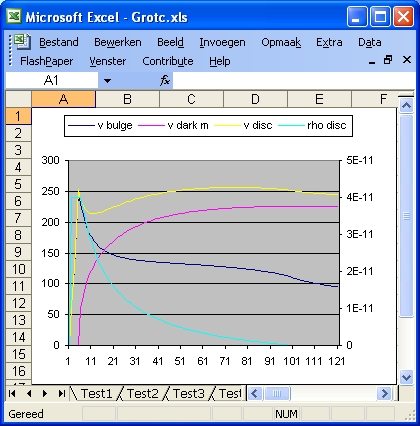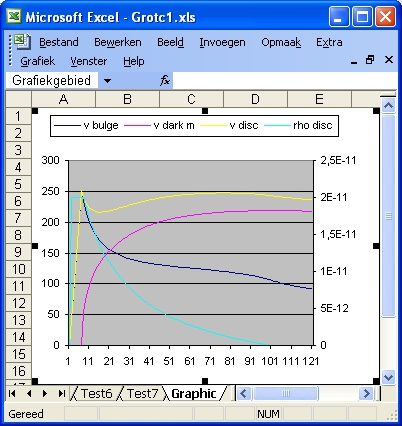## Description of Excel program: Grotc.xls and Grotc1.xls

The purpose of the Excel programs is to calculate your own galaxy rotation curve as a function of a bulge, a disk and a halo of darkmatter. This halo is an option
This procedure goes in two steps:
• First you enter the parameters with the dark matter parameters equal to zero, and then you observe the galaxy rotation curve. This rotation represents the expected curve based on visible mass. The strategy should be that the rotation curve matches the visible mass.
• Secondly you change the dark matter parameters and you observe the galaxy rotation curve. The strategy should be that the rotation curve becomes flat. This rotation curve represents the observed curve. If not you try again.
There are two dark matter profiles considered: NFW and Hernquist
For a copy of the programs in zip format select grotc.xls.zip This file also contains the programs Grotcexp.xls and Grotcesxp1.xls. For more details of both See: grotcexp.xls
The main physical assumption is that visible matter and darkmatter behave similar. In this simulation for both visible matter and dark matter this is Newton's Law
The main problem or chalenge is not to include to much darkmatter, at least such that the darkmatter density should not be higher than the visible matter, because otherwise it will become visible.

In Excel the distance of the galaxy rotation curve is expressed in Units. One unit is identical with one line of information (results) and is equivalent with 1000 Lightyears.
The average radius of a rotation curve is 100 units or 100000 Lightyears or 30 kpc.
The standard distance of the bulge is 6 units or 6000 Lightyears. This distance is defined by the parameter rbulge. Within this range the speed of the rotation curve increases lineair to 250 km/sec. There after the speed is calculated as a function of the density.
The program consists of approximate 6 tests: Test1, Test2, Test3, Test4, Test5 and Test6
Each test has 11 parameters: "NFW", "r bulge", "r disk", "rdisp", "rs" , "rhos", "a", "Mh", "rho disk str", "rho disk end" and "Filter"

• The variable "NFW" (or "Hernquist") is used to select the NFW profile. When NFW is 1 the NFW profile is selected. When NFW is 0 the Hernquist profile is selected. When this is the case the description changes into "Hernquist" and the value into 1. When Hernquist is 0 the reverse happens.
• The variable "r bulge" shows the length of the bulge in Lightyears. The maximum value is 100000.
• The variable "r disk" shows the length of the disk in Lightyears. The maximum value is 400000.
• The variable "r disp" shows the length of the disk in Lightyears. The maximum value is 400000.
• "rs" and "rhos" are two parameters of the NFW dark mattter profile. The dimension of rs is in Lightyears. See http://lanl.arXiv.org/pdf/astro-ph/0301144 page 17 for more details.
• "a" and "Mh" are two parameters of the Hernquist dark mattter profile. The dimension of "a" is in Lightyears. The dimension of Mh is in 10^12*m0, with m0 being the mass of the Sun. See http://lanl.arXiv.org/pdf/astro-ph/0506015 page 2 for more details.
• "rho disk start", "rho disk end" and "filter" are input parameters to calculate the disk profile. See below

In order to start the simulation select START button.

Each simulation consists only of 1 phase. In effect this phase is the same as phase 4 of the Excel programs Circ11.xls, Circ12.xls etc.

In this phase the galaxy rotation curve is calculated as a function of the density of the bulge, the disk and the density of dark matter profile (either NFW or Hernquist).
In the programs Circ11, Circ12 etc the density profile of the disk was calculated based starting from a flat rotation curve (if VSEL = 1). For a description of that program select circ11.xls.htm
In this program the density is calculated based on the three parameters:

rhodisks (rho disk start), rhodiske (rho disk end) and filter
• When r <= rbulge then rho = (v/rbulge)^2 * (3*G/4*pi) with v(rbulge) = 250 km/sec
In this range rho is constant because v(r)/r is also constant.
When rhodisks = 0 then rhodisks = rho.
• when r > rbulge with the following formula :
rhob=rhodisks - rhodiske
rho = rhob * filter / (filter + r - rbulge - n) - rhob * filter / (filter + rdisk - rbulge - n) + rhodiske
The parameter n is calculated such that when r is equal to rbulge + 1, rho is equal to rhodisks. For an exponential disk shape See: grotcexp.xls

For readers not familiar with rotation curves select this overview article by Stephen M. Kent: http://adsbit.harvard.edu/cgi-bin/nph-iarticle_query?bibcode=1987AJ.....93..816K
There is also a PDF version available from that link. Rememember that Andromeda galaxy, M31 and N224 are one and the same.

• ### Program Grotc.xls - NFW Profile

#### Test1, Test2 and Test3

Those 3 tests belong together. There is no dark matter included. The only difference is the parameter "rho disk start" i.e. the density value of the disk immediate when the bulge ends and the disk starts. In order to make the rotation curve smoothly it is assumed that there is also a disk mass inside the bulge.
• The purpose of these tests (including test4) is to find the galaxy rotation curve based on visible mass.
• In Test1 the parameter "rho disk start" is initially set to zero (On purpose). When that is the case the starting value is calculated and set equal to the density of the bulge i.e. "rho bulge" in column "D".
• In Test2 "rho disk start" is set equal to: "1.2E-10", in Test3 to: "6E-11" and in Test4 to: "2E-11".
• the parameter mass b shows the mass of the bulge. The parameter mass disk shows the mass of the disk.
The total galaxy mass values are: 43E+40, 34E+40, 19E+40 and 8.6E+40.
• The shape of Test1 resembles a flat galaxy rotation curve. Test 4 simulates a galaxy rotation curve based on visible mass (luminosity).
• What Test4 indicates, relative to Test1, that roughly a factor 4 is missing.

#### Test4, Test5, Test6 and Test7

Those four tests are used to find the amount of dark matter described by the NFW profile necessary to support a flat galaxy rotation curve . They belong together because the "rho disk start" values are the same (2E-11)Test 7 - NFW profile

### Parameters of Excel program Grotc.xls

 Parameter Test1 Test2 Test3 Test4 Test5 Test6 Test7 1 NFW 1 1 1 1 1 1 1 2 rbulge 4000 4000 4000 4000 4000 4000 4000 3 disk 100000 100000 100000 100000 100000 100000 100000 4 NFW rs 0 0 0 7500 15000 30000 48000 5 NFW rhos 0 0 0 60E-12 15E-12 3,5E-12 1,35E-12 6 Hq a 0 0 0 0 0 0 0 7 Hq MHalo 0 0 0 0 0 0 0 8 Rho disk s 15.6E-11 12E-11 6E-11 2E-11 2E-11 2E-11 2E-11 9 Rho disk e 0,0 0,0 0,0 0,0 0,0 0,0 0,0 10 filter 15 15 15 15 15 15 15 11 Rho NFW 0 0 0 150E-13 25E-13 8,75E-13 3,375E-13 12 tot m bulge 3,54 3,54 3,54 3,54 3,54 3,54 3,54 13 tot m disk 39,57 30,4 15,2 5,07 5,07 5,07 5,07 14 tot m dm 0.0 0.0 0.0 274,01 506,63 943,19 1490,5 15 max r dm 0 0 0 291714K 538547K 1005284K 1588234K

### Program Grotc1.xls - Hernquist Profile

#### Test1, Test2 and Test3

Those 3 tests belong together. There is no dark matter included. The only difference is the parameter "rho disk start" i.e. the density value of the disk immediate when the bulge ends and the disk starts. In order to make the rotation curve smoothly it is assumed that there is also a disk mass inside the bulge.
• The purpose of these tests is to find the expected galaxy rotation curve based on visible mass.
• In Test1 the "rho disk start" is zero. When that is the case the starting value is equal to the density of the bulge i.e. "rho bulge" in column "D".
• The different starting values are: "6.94-11", "4E-11" and "2E-11"
• the parameter mass b shows the mass of the bulge. The parameter mass disk shows the mass of the disk.
The total galaxy mass values are: 31E+40, 20E+40 and 13E+40.
• Test 3 simulates a galaxy rotation curve based on visible mass (luminosity).

#### Test4, Test5, Test6, and Test7

Those four tests are used to find the amount of dark matter described by the Hernquist profile necessary to support a flat galaxy rotation curve . They belong together because the "rho disk start" values are the same (2E-11)Test 7 - Hernquist profile

### Parameters of Excel programs Grotc1.xls

 Parameter Test1 Test2 Test3 Test4 Test5 Test6 Test7 1 Hernquist 1 1 1 1 1 1 1 2 rbulge 4000 4000 4000 4000 4000 4000 4000 3 rdisk 100000 100000 100000 100000 100000 100000 100000 4 NFW rs 0 0 0 0 0 0 0 5 NFW rhos 0 0 0 0 0 0 0 6 Hq a 0 0 0 15000 30000 60000 96000 7 Hq MHalo 0 0 0 0,27 0,5 0,9 1,35 8 Rho disk s 15,64E-11 12E-11 6E-11 2E-11 2E-11 2E-11 2E-11 9 Rho disk e 0,0 0,0 0,0 0,0 0,0 0,0 0,0 10 filter 15 15 15 15 15 15 15 11 Rho NFW 0 0 0 0 0 0 0 12 tot m bulge 3,55 3,55 3,55 3,55 3,55 3,55 3,55 13 tot m disk 39,6 30,4 15,2 5,07 5,07 5,07 5,07 14 tot m dm 0.0 0.0 0.0 45 95,1 175 265 15 max r dm 0.0 0.0 0.0 3850K 7701K 14607K 22624K

#### Discussion

Test3 and Test4 of Grotc.xls and Test2 and Test3 of Grotc1.xls are all examples that dark matter is included.
What those tests tell you is
• that it is not so difficult, when using either the NFW profile or the Hernquist profile, to create with dark matter flat galaxy rotation curves.
• that the amount of dark matter for the Hernquist profile is much less as for the NFW profile. The Hernquist profile is from 1990 and the NFW profile is from 1994. This is interesting because you would expect that the latest profile would result in less dark matter. But this is wrong, the reverse is true.
Test1 and Test7 of Grotc.xls are very interesting from the point of view of visible matter. The same for Test6 and Test7 of Grotc1. Test7 of Grotc.xls and Test7 of Grotc1.xls are the same.
• They teach you if you want to go from visible rotation curve (Test1) to a flat rotation curve (Test7) the extra amount of visible matter required is only a factor 2 (2856 versus 6176). With darkmatter (Hernquist profile a=40 Mh=10000) the amount of dark matter required is 9417. That means an increase with a factor 4.5 compared to the disk mass.
• You can also do a slightly different test. This is Test6 of Grotc1.xls. In Test6 r disk is 50 and half the size of Test7 i.e. 100. In Test6 rho disk e to 0.03. What you will get is a rotation curve of roughly half the size of Test7. Mass disk = 955.06 (Compared to 2035 for Test7). That means if you what to stretch a rotation curve with a factor 2, the extra amount of disk mass required is roughly the same as the original.

Created: 23 November 2005